# Dynamical systems (Part - 5) - Classical Mechanics, UGC - NET Physics Physics Notes | EduRev

## Physics : Dynamical systems (Part - 5) - Classical Mechanics, UGC - NET Physics Physics Notes | EduRev

The document Dynamical systems (Part - 5) - Classical Mechanics, UGC - NET Physics Physics Notes | EduRev is a part of the Physics Course Physics for IIT JAM, UGC - NET, CSIR NET.
All you need of Physics at this link: Physics

Examples

Example 1

Consider linear dynamical system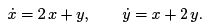There is only one critical point at the origin,

xC = 0, yC = 0.

Since the system is linear, we do not have to linearize it and can write the matrix of linearized system immediately: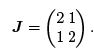Its eigenvalues are calculated from (8.27):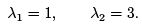Eigenvectors can be found easily by hand. Recall that eigenvectors are solutions to equation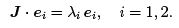(Notice that we suppress the Einstein summation convention). For i = 1 we obtain homogeneous system of linear equations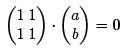where e1 = (a, b) is unknown eigenvector. Since the rows (or columns) of the matrix above are linearly dependent, this system has infinitely many non-trivial solutions satisfying condition a = -b. Hence, all eigenvectors corresponding to eigenvalue λ1 = 1 have the form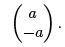We choose the eigenvector to be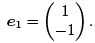By similar consideration we nd that the eigenvector associated with eigenvalue λ2 = 3 is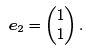To summarize, we have found the eigensystem of matrix J :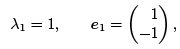(8.28)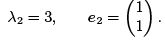Now we can classify the critical point (0, 0). Since the eigenvalues are real and nonzero, critical point is hyperbolic. They are both positive and hence the critical point is unstable node. Finally, eigenvectors are real and so the system has two unstable manifolds given by e1 and e2. Implementation in Mathematica is shown in figure 8.11.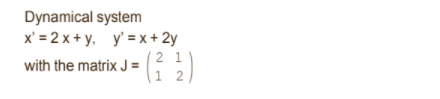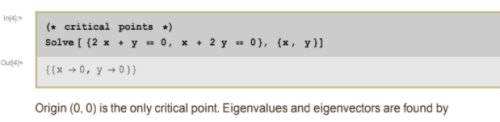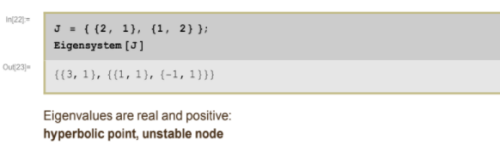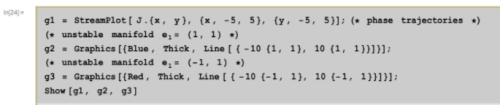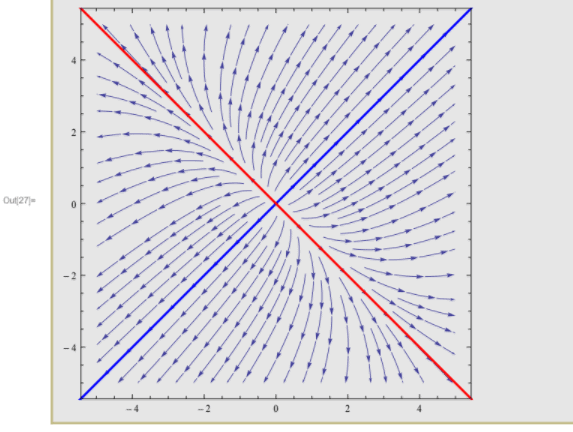Fig. 8.11. Example 1.

Example 2

Linear dynamical system has the form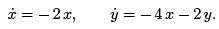The matrix of this system is clearly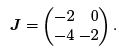Eigenvalues are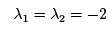and so it is a degenerate node. Since both eigenvalues are negative, it is a stable degenerate node. Implementation in Mathematica is shown in figure 8.12.

Example 3. Volterra-Lotka equations

Volterra-Lotka equations belong to the class of predator-prey models which describe interaction between two populations. The population of preys has a tendency to grow and the population of predators tends to die. It is due to their mutual interaction that also the population of predators can grow and the population of preys can die, in other words, predators are eating preys.

Let x = x(t) be the number of preys, say, rabbits, let y = y(t) be the number of predators, say, foxes. We can construct a plausible model of interaction between foxes and rabbits by following simple considerations. Suppose that y = 0, i.e. there are only rabbits present. As a rst approximation we can assume that the population of rabbits will grow, the number of rabbits x will increase because of "interaction" between rabbits and the higher is the number of rabbits, the higher is the rate of growth. Hence, we can postulate that isolated population of rabbits will be governed by equation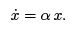Roughly speaking, constant α can be interpreted as a probability of the birth of a new rabbit when there are no foxes. This equation has solution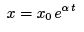which means that isolated population of rabbits will grow exponentially.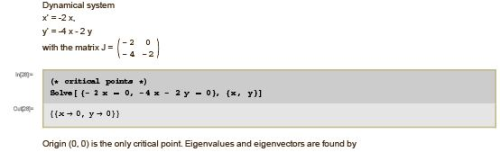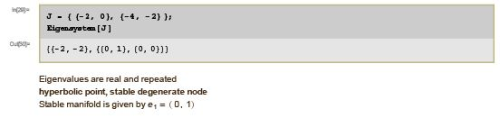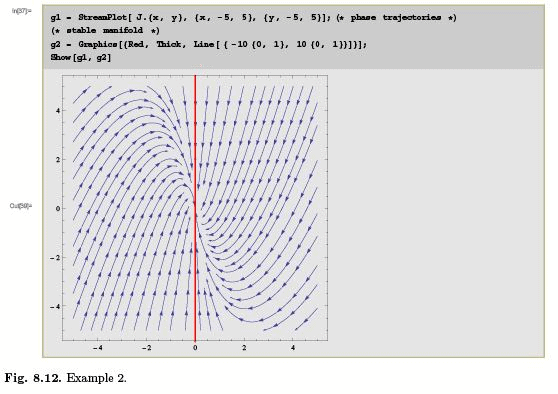Similar consideration applies to isolated population of foxes. If γ is the probability of death of the fox, isolated population of foxes will be governed by equation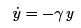which has the solution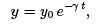i.e. the population of foxes will die exponentially.
Now we add an interaction to our equations. The number of rabbits eaten by foxes is proportional to number of rabbits and to number of foxes. Conversely, the number of new-born foxes is proportional to number of foxes and to number of rabbits. If we introduce constants β and δ for both processes, equations for interacting populations of rabbits and foxes read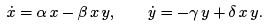(8.29)

These are Volterra-Lotka equations. Obviously, they are non-linear and the nonlinearity represents the interaction between two populations. All constants are assumed to be positive.

Critical points can be found by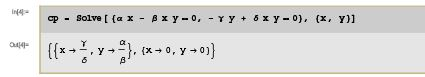Hence, the critical points are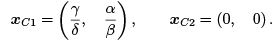In order to linearize equations (8.29) we introduce the Jacobi matrix J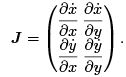The Jacobi matrix can be found in Mathematica by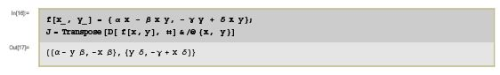which shows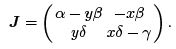Next we evaluate the Jacobian at both critical points: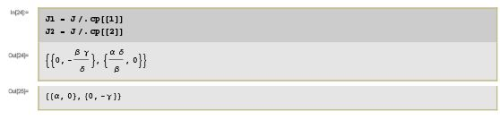i.e. we have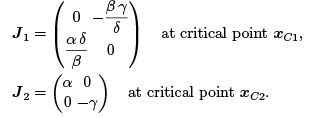Finally we nd eigenvalues and eigenvectors by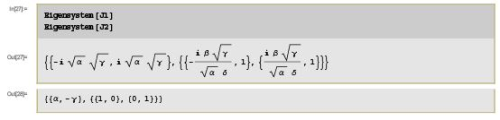Flow of the vector field

In this section we introduce some useful notions related to the concept of dynamical system. We consider general autonomous dynamical system (8.1)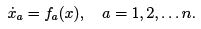(8.30)

We know that the solution exists and is unique if prescribe initial conditions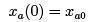(8.31)

where xa0 are constants with the meaning of initial value of coordinates xa. The solution of dynamical system is then a set of functions xa as functions of time,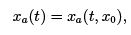(8.32)

where we have explicitly emphasized that particular solution depends on initial values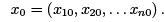Hence, in the following, by symbol x(t; x0) we mean the set of functions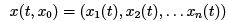such that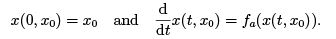(8.33)

In other words, x(t, x0) is a solution of dynamical system (8.30) with initial conditions (8.31).
It is useful to introduce slightly more formal notation for x(t, x0). We de ned the phase space M as an abstract space with coordinates xa. For n-dimensional dynamical system, the phase space is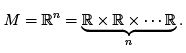The flow of dynamical system (8.30) is a mapping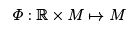defined by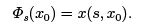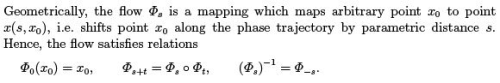Obviously,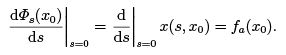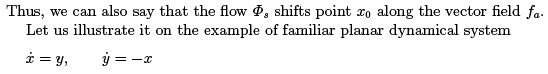so that we have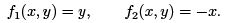Vector field fa can be plotted by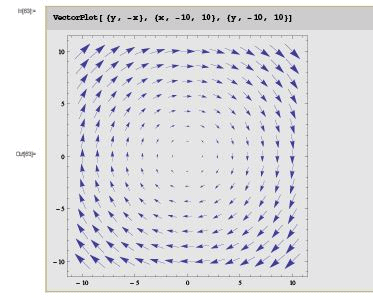This dynamical system for initial conditions

x(0) = x0, y(0) = y0, can be solved explicitly by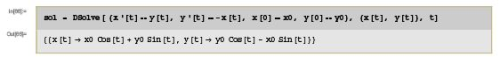which shows, in the notation introduced above,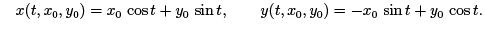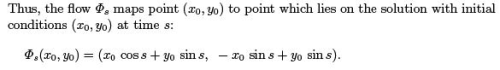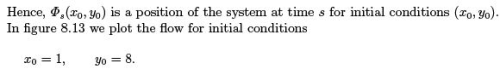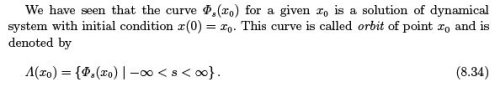Similarly, we de ne positive semi-orbit and negative semi-orbit by(8.35)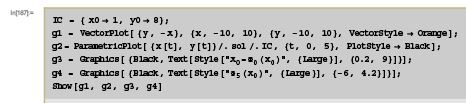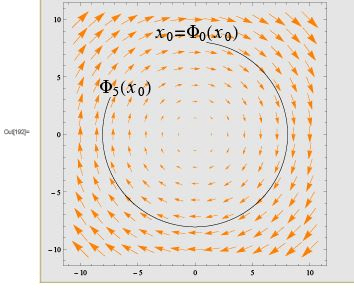Fig. 8.13. Illustration of the flow.

Lyapunov stability

Recall that we have de ned the critical point or fixed point xof dynamical system (8.30) as such point xC for which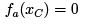and hence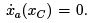System with initial conditions x0 = xC is in equilibrium in the sense that it remains in the critical point at all times, i.e.

∧(xC ) = {x}

In other words, critical point xC satis es relation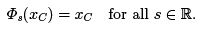We have classi ed critical points according to behaviour of the orbits (phase trajectories) in the vicinity of the critical point. If the orbit remained in the vicinity of critical point, we have said that the critical point is stable. If the orbit was attracted to critical point, it was called stable node or stable focus, depending on the character of the system. If the orbit was circular, critical point was called centre. Finally, if the orbit escaped from the critical point to in nity, we called the critical point the unstable node or unstable focus. However, this analysis was performed for linearized dynamical system. Now we can formulate the stability for general non-linear system in terms of the
ow.

Let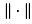be standard norm de ned on the phase space M , i.e. for any x ∈ M its norm is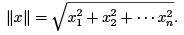In general, the norm is a measure of distance of point x from the origin. In some situations, it is useful to introduce different notion of the norm, for example the so-called p norm (p is positive integer) de ned by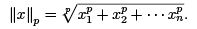In the following we will use standard norm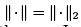which is a standard Euclidean distance, as follows from the Pythagorean theorem. In general, the norm must satisfy three relations.

• Positive definiteness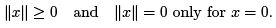• Linearity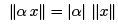for arbitrary real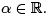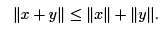In some contexts the first condition is relaxed, i.e. we admit there are vectors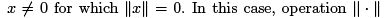is called semi-norm. In this textbook we consider only positive definite norms satisfying the first property. Notice that positive definiteness implies that whenever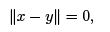vectors x and y are equal, x = y.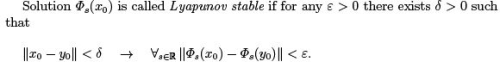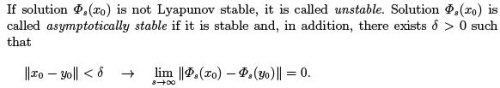Offer running on EduRev: Apply code STAYHOME200 to get INR 200 off on our premium plan EduRev Infinity!

159 docs

,

,

,

,

,

,

,

,

,

,

,

,

,

,

,

,

,

,

,

,

,

,

,

,

;